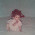### ROLLUP Function

The teradata ROLLUP function is used, when ever an aggregation is required across all levels of a hierarchy within a single dimension.

Lets take geography is a dimension. You might have 3 levels of hierarchy - city, county and state. You may wish to produce sum of revenue amounts across all three levels.

we can produce below samples
• Produce a sum of revenue amounts across all three levels.
• Aggregate at the lowest level (city) and 'rollup' those aggregations to the next level (county) and finally rollup to the top level (state).
• Provide a grand total across all three levels.
All of these are easily done with the ROLLUP feature.

Example1:
Using the GROUP BY function, produce a total of salaries by department for department numbers less than 402.

SELECT department_number
,SUM(salary_amount)
FROM employee WHERE department_number < 402
GROUP BY 1
ORDER BY 1;

 department_number Group Sum(salary_amount) 100 100000 201 73450 301 116400 302 56500 401 245575

ROLLUP Function - One Level
Using the ROLLUP function, produce salary totals by department.

SELECT department_number
,SUM(salary_amount)
FROM employee
WHERE department_number < 402
GROUP BY ROLLUP (department_number)
ORDER BY 1;

 department_number Group Sum(salary_amount) ? 591925 100 100000 201 73450 301 116400 302 56500 401 245575

There is an additional row with a '?' department number. This row represents the rollup of all department salaries which is the top (and only) level of the hierarchy in this case. The '?' does not represent a null, rather it represents the 'total' of all department salaries.

ROLLUP Function - Two Levels

Produce a hierarchy of total salaries by department within manager.

SELECT manager_employee_number AS Mgr
,department_number AS Dept
,SUM(salary_amount) AS SumSal
FROM employee
WHERE department_number < 402
GROUP BY ROLLUP (manager_employee_number,department_number)
ORDER BY 1,2;

 Mgr Dept SumSal ? ? 591925 (all mgrs,all depts,all emps) 801 ? 286750 (mgr 801,all depts, 5 emps) 801 100 100000 (mgr 801,dept 100, 1 emp) 801 201 34700 (mgr 801,dept 201, 1 emp) 801 301 57700 (mgr 801,dept 301, 1 emp) 801 302 56500 (mgr 801,dept 302, 1 emp) 801 401 37850 (mgr 801,dept 401, 1 emp) 1003 ? 207725 (mgr 1003,all depts, 6 emps) 1003 401 207725 (mgr 1003,dept 401, 6 emps) 1019 ? 58700 (mgr 1019,all depts, 2 emps) 1019 301 58700 (mgr 1019,dept 301, 2 emps) 1025 ? 38750 (mgr 1025,all depts, 1 emp) 1025 201 38750 (mgr 1025,dept 201, 1 emp)

Three separate aggregations taking place in the preceding query.

Totals for each dept/manager combo (black).
This is represented as the set '(manager,department )' and is the bottom of the hierarchy.

Totals for each manager across all departments (red).
This is represented as the set '(manager )' which is the middle of the hierarchy.

A total for all depts across all managers (blue).
This is represented as the set '( )' and is the top of the hierarchy.

ROLLUP WITH NULL
As previously indicated, the row with the '?' represents the grand total of all salaries across all departments.
But what if there was a department number with a null? How would we distinguish between it and a 'total' row?

Add an employee with a null department and a null job code:

INSERT INTO employee VALUES
(1050,801,NULL,NULL,'LaCoste','Jason',780415,480816,60000.00);

SELECT department_number
,SUM(salary_amount)
FROM employee
GROUP BY ROLLUP (department_number)
ORDER BY 1;

 department_number Group Sum(salary_amount) ? 651925 ? 60000 100 100000 201 73450 301 116400 302 56500 401 245575

While it may seem obvious that the row with the larger sum will represent the 'total' row,
a function exists which permits the report to be more easily read.

#### 4 comments:

1.nice example sir.

1.Thank you so much Bhaskara

2.Excellent explanation...

3.very well explained.Thank you